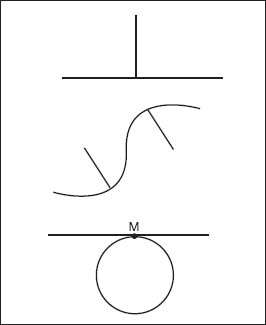NextPrevious

# What does the term bisect mean?

The term “bisect” is important in geometry; it means to cut into two (or divide into half), mainly lines (or two-dimensional figures) and angles. To compare, a bisected line segment means finding the plane, line, or point that is the midpoint of the line segment. This is also called a segment bisector. An angle bisector is a ray in the interior of an angle that forms two equal angles. First locate the point on each ray that is equally distant from the vertex; then draw a third point equally distant from each of the first two rays. A line that extends through the third point and the vertex is the angle bisector.

To draw a bisector, follow the following sequence (as per the illustration): First, draw an angle; then draw an arc centered at the vertex (endpoint), in which B and C are the intersections of the arc and angle lines at equal distances from the vertex; next draw an arc centered at B and one centered at C—both with the same radii—inside the angle; finally, extend a line from the vertex to the point D where the arcs of B and C intersect—making AD the bisector of the angle at A.Examples of a perpendicular line (top), normal lines (middle), and tangential lines (bottom), with point M being the point of tangency.

Close

This is a web preview of the "The Handy Math Answer Book" app. Many features only work on your mobile device. If you like what you see, we hope you will consider buying. Get the App